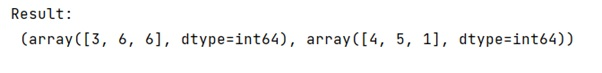# What is an intuitive explanation of numpy.unravel_index()?

Learn about the Python's numpy.unravel_index() method with examples.
Submitted by Pranit Sharma, on January 17, 2023

NumPy is an abbreviated form of Numerical Python. It is used for different types of scientific operations in python. Numpy is a vast library in python which is used for almost every kind of scientific or mathematical operation. It is itself an array which is a collection of various methods and functions for processing the arrays.

## Python - numpy.unravel_index() Method

Computer memory is addressed linearly. Each memory cell corresponds to a number. A block of memory can be addressed in terms of a base, which is the memory address of its first element, and the item index.

This method converts a flat index or array of flat indices into a tuple of coordinate arrays.

Syntax:

```numpy.unravel_index(indices, shape, order='C')
```

Parameter(s):

• indices: array_like - An integer array whose elements are indices into the flattened version of an array of dimensions shape. Before version 1.6.0, this function accepted just one index value.
• shape: tuple of ints - The shape of the array to use for unraveling indices.
• order: {'C', 'F'}, optional - Determines whether the indices should be viewed as indexing in row-major (C-style) or column-major (Fortran-style) order.

Let's understand with the help of an example,

## Python code to demonstrate the example of numpy.unravel_index()

```# Import numpy
import numpy as np

# Creating a numpy array
arr = np.array([22, 41, 37])

# Using unravel index
res =  np.unravel_index(arr,(7,6))

# Display result
print("Result:\n",res)
```

Output:What's New (MCQs)

Top Interview Coding Problems/Challenges!

IncludeHelp's Blogs

Languages: » C » C++ » C++ STL » Java » Data Structure » C#.Net » Android » Kotlin » SQL
Web Technologies: » PHP » Python » JavaScript » CSS » Ajax » Node.js » Web programming/HTML
Solved programs: » C » C++ » DS » Java » C#
Aptitude que. & ans.: » C » C++ » Java » DBMS
Interview que. & ans.: » C » Embedded C » Java » SEO » HR
CS Subjects: » CS Basics » O.S. » Networks » DBMS » Embedded Systems » Cloud Computing
» Machine learning » CS Organizations » Linux » DOS
More: » Articles » Puzzles » News/Updates